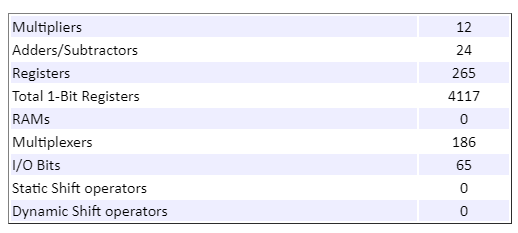# Optimize Programmable FIR Filter Resources

This example shows how to use programmable coefficients with the Discrete FIR Filter block and how to optimize hardware resources for programmable filters.

The Discrete FIR Filter block optimizes resource use when the filter coefficients are symmetric or antisymmetric or are zero-valued. To use these optimizations with programmable coefficients, all of the input coefficient vectors must have the same symmetry and zero-valued coefficient locations. Set the Coefficients prototype parameter to a representative coefficient vector. The block uses the prototype to optimize the filter by sharing multipliers for symmetric or antisymmetric coefficients, and removing multipliers for zero-valued coefficients.

If your coefficients are unknown or not expected to share symmetry or zero-valued locations, set Coefficients prototype to `[]`. When you do so, the block does not optimize multipliers.

This example shows how to set a prototype and specify programmable coefficients for a symmetric filter and a filter with zero-valued coefficients. The example also explains how the block reduces the number of multipliers in the filter in these cases.

### Symmetric Filter Coefficients

Design two FIR filters, one with a lowpass response and the other with the complementary highpass response. Both filters are odd-symmetric and have 43 taps.

```Fpass = 0.45; % Passband frequency Fstop = 0.55; % Stopband frequency Apass = 1; % Passband attenuation (dB) Astop = 60; % Stopband attenuation (dB) f = fdesign.lowpass('Fp,Fst,Ap,Ast',Fpass,Fstop,Apass,Astop); Hlp = design(f,'equiripple','FilterStructure','dffir'); % Lowpass Hhp = firlp2hp(Hlp); % Highpass hpNumerator = Hlp.Numerator; %#ok<NASGU> lpNumerator = Hhp.Numerator; %#ok<NASGU> ```

The example model shows a filter subsystem with a control signal to switch between two sets of coefficients. The HDL Algorithm subsystem includes a Discrete FIR Filter block and the two sets of coefficients defined by the created workspace variables.

```modelname = 'ProgFIRHDLOptim'; open_system(modelname); ```Because two sets of coefficients are symmetric in the same way, you can reduce the number of multipliers in the filter implementation by setting the Coefficient prototype parameter of the Discrete FIR Filter block. Set Coefficient prototype to either of the coefficient vectors. The example model sets the prototype to `hpNumerator`.

When you use the prototype for symmetric coefficients, provide only the unique coefficients to the coeff port. In this case, the filter has 43 odd-symmetric coefficients, so the input port expects the first half of the coefficients, that is, 22 values.

```open_system('ProgFIRHDLOptim/HDL Algorithm'); ```The model switches coefficients every 100 cycles. The filtered output data shows the effect of the lowpass and highpass coefficients.

```T = 512; sim(modelname); ```The model is configured to output the resource report from HDL code generation. This feature enables you to see the number of multipliers in the filter implementation. Because the block shares multipliers for symmetric coefficients, the filter implementation uses 22 multipliers rather than 43.### Zero-Valued Filter Coefficients

Design two halfband FIR filters, one with a lowpass response and the other with the complementary highpass response. Both filters have 43 symmetric taps, where every second tap is zero. Set the Coefficient prototype parameter to either of the coefficient vectors. Changing the workspace value of `hpNumerator` updates the prototype in the block.

Similarly, specify 22 coefficients at the input port. Although no multipliers exist for the zero-valued coefficients, for the block to maintain the correct alignment of the coefficients, you must specify the zero-valued coefficients at the port.

```N = 42; f = fdesign.halfband('N,Ast',N,Astop); Hlp = design(f,'equiripple','FilterStructure','dffir'); % Lowpass Hhp = firlp2hp(Hlp); % Highpass hpNumerator = Hlp.Numerator; lpNumerator = Hhp.Numerator; sim(modelname); ```The model is configured to output the resource report from HDL code generation. This feature enables you to see the number of multipliers in the filter implementation. In this case, because the filter optimizes for symmetry and zero-valued coefficients, the implementation uses 12 multipliers rather than 43.